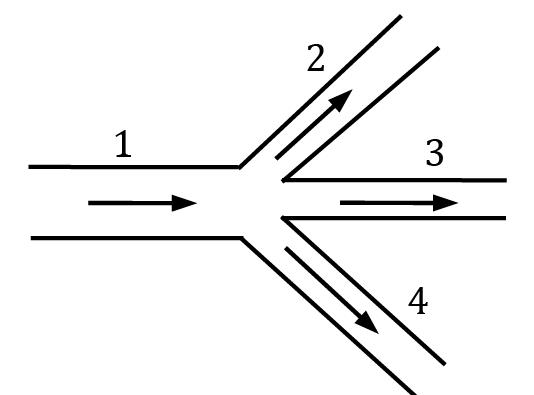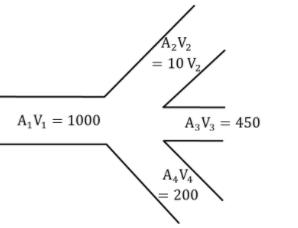Courses

# Test: Fluid Kinematics Level - 1

## 15 Questions MCQ Test Engineering Mechanics | Test: Fluid Kinematics Level - 1

Description
This mock test of Test: Fluid Kinematics Level - 1 for Civil Engineering (CE) helps you for every Civil Engineering (CE) entrance exam. This contains 15 Multiple Choice Questions for Civil Engineering (CE) Test: Fluid Kinematics Level - 1 (mcq) to study with solutions a complete question bank. The solved questions answers in this Test: Fluid Kinematics Level - 1 quiz give you a good mix of easy questions and tough questions. Civil Engineering (CE) students definitely take this Test: Fluid Kinematics Level - 1 exercise for a better result in the exam. You can find other Test: Fluid Kinematics Level - 1 extra questions, long questions & short questions for Civil Engineering (CE) on EduRev as well by searching above.
QUESTION: 1

### A steady, incompressible, two–dimensional velocity field is given by V‾ = (u, v) = (0.5 + 0.8x)î + (1.5 − 0.8 y)ĵ The number of stagnation points in the flow field is

Solution: Stagnation points are those points where (u, v) = 0

u = 0.5 + 0.8x = 0

∴ x = −0.5 / 0.8 = −0.625

v = 1.5 − 0.8y = 0

∴ y = 1.5 / 0.8 = 1.875

i.e there is only one stagnation point at (−0.625, 1.875)

QUESTION: 2

### A stream function is given by ψ = 4x − 3y. The magnitude of resultant velocity at any point is

Solution: Given, ψ = 4x − 3y

As per definition of stream function

u = ∂ψ / ∂y and v = −∂ψ / ∂x

∴ u = −3 & v = −4 So V- = −3î − 4ĵ

and magnitude of resultant velocity is given |⃗V⃗ | = √u2 + v2 = 5

QUESTION: 3

### Match List-I (Pipe flow) with List-II (Type of acceleration) and select the correct answer.List-I List-IIa. Flow at constant rate through a bend 1. Zero accelerationb. Flow at constant rate through a 2. Local and convective accelerationstraight uniform diameter pipec. Gradually changing flow through a bend 3. Convective accelerationd. Gradually changing flow through a straight pipe 4. Local accelerationCodes:a b c d

Solution: For flow through bends, the direction of velocity changes as the fluid moves through the bend but since the flow rate is constant, and if cross-section is uniform the flow is steady, hence only convective acceleration exists.

Similarly for constant flow rate through a straight, uniform diameter pipe, there is no change of velocity, (neither magnitude nor direction) so total acceleration is zero.

For a flow through bend where the discharge is not constant, the velocity changes both due to geometry of pipe and with time, so both local and convective acceleration exist.

For variable discharge through a straight pipe, the velocity will change with time, but it will be same across the whole pipe at any particular time instant, i.e the flow is unsteady but uniform, only local acceleration exists.

QUESTION: 4

Which one of the following stream functions represents a possible irrotational flow field?

Solution: For irrotational flow, stream function must satisfy Laplace equation i.e

∂2ψ / ∂x2 + ∂2ψ / ∂y2 = 0

Checking all the given options, we find option (A) satisfies the equation.

QUESTION: 5

The velocity potential function for a two dimensional flow field is given by ϕ = x2 − y2. The magnitude of velocity at the point (1,1) is

Solution: ϕ = x2 − y2

we know u = −∂ϕ / ∂x and v = −∂ϕ / ∂y

∴ u = −(2x), v = −(−2y)

⇒ u = −2x, v = 2y

Thus at (1, 1),⃗V⃗ = −2î + 2ĵ |⃗V⃗ | = √4 + 4 = 2 × 21/2

QUESTION: 6

Pipe 1, branches into three pipes as shown in the given figure. The areas and corresponding velocities are as given in the following table the value of V2 in cm per second will bePipe Velocity (cm per second) Area (sq. cm) 1. 50 20 2. V2 10 3. 30 15 4. 20 10

Solution:For incompressible fluid, A1V1 = A2V2 + A3V3 + A4V4

1000 = 10 V2 + 450 + 200

V2 = 35 cm/s

QUESTION: 7

If the stream function for a 2D flow is given as ψ = 3xy, then the velocity at the point (2, 3) will be

Solution: Given, ψ = 3xy By definition of ψ

u = ∂ψ / ∂y and v = −∂ψ / ∂x

So u = 3x, v = −3y

at point (2, 3)

⃗V = 6î − 9ĵ and |⃗V⃗ | = √62 + 92 = 10.816

≈ 10.82 Units

QUESTION: 8

If the velocity potential function ϕ for a flow satisfies the Laplace equation δ2ϕ⁄δx2 + δ2ϕ⁄δy2 + δ2ϕ⁄δz2 = 0 then the flow is

Solution: Velocity potential function is defined only for irrotational flows, whether the flow is steady or unsteady, compressible or incompressible. If it also satisfies Laplace equation then the flow is also incompressible.

QUESTION: 9

Circulation is defined as the line integral of tangential component of velocity along a

Solution: Circulation is defined as the cyclic integral of tangential velocity about a closed loop.

QUESTION: 10

Which of the following flow is rotational? (where u and v are velocity components in x and y directions respectively)

Solution: For a 2D flow to be rotational

Ωz = 0

Checking option (A)

Ωz = ∂v / ∂x −∂u / ∂y =3/2 − 1 ≠ 0

Checking option (B)

Ωz = ∂v / ∂x −∂u / ∂y = 2xy − 2xy = 0

QUESTION: 11

For irrotational flow, the curl of velocity vector is

Solution: The curl of velocity vector represents the vorticity of flow, and for irrotational flow, the vorticity is zero. i.e curl of velocity = 0 for irrotational flow.

QUESTION: 12

A flow field satisfying ⛛.V‾= 0 as the continuity equation represents

Solution: For general flow, ∂ρ / ∂t + V- ∙ (ρV ) = 0 ⋯ ①

If ρ = C

i. e. ρ ≠ ρ(x, y, z, t)

Hence equation ① is reduced to V ∙⃗V = 0 for incompressible flow.

QUESTION: 13

A steady incompressible 2D flow field is given by u = 2x2 + y2 and v = −4xy. The convective acceleration along x-direction at point (1, 2) is

Solution: u = 2x2 + y2, v = −4xy

ax = (2x2 + y2)(4x) + (−4xy)(2y) + 0 + 0

∴ at point (1, 2) ax

= (2 + 4)(4) − (8)(4) ax

= 24 − 32 ax

= −8 units

QUESTION: 14

A two-dimensional flow field is defined as V‾ = xî − yĵ. The equation of the streamline passing through the point (1, 2) is

Solution: V = xî − yĵ

i. e u = x, v = −y

For a streamline in 2D flow,

dy / dx =v / u ⟹ dy / dx =−y /x

⇒ ln y = ln 1 / x + C

at (1, 2) ln 2 = ln 1 + C

C = ln 2

So, ln y = ln 2 / x

or xy = 2 or xy − 2 = 0

QUESTION: 15

In a two dimensional flow in x-y plane, if ∂u / ∂y = ∂v / ∂x , then the fluid element will undergo

Solution: For a 2D planar flow the deformation rate is given as ε̇ xy = ∂v / ∂x + ∂u / ∂y And rotation rate is given as

ωz = 1/[∂v / ∂x − ∂u / ∂y ]

Therefore in the given case ε̇ xy = 2 ∂u / ∂y and ωz = 0

Thus along with translation, elements will deform but shall not rotate.